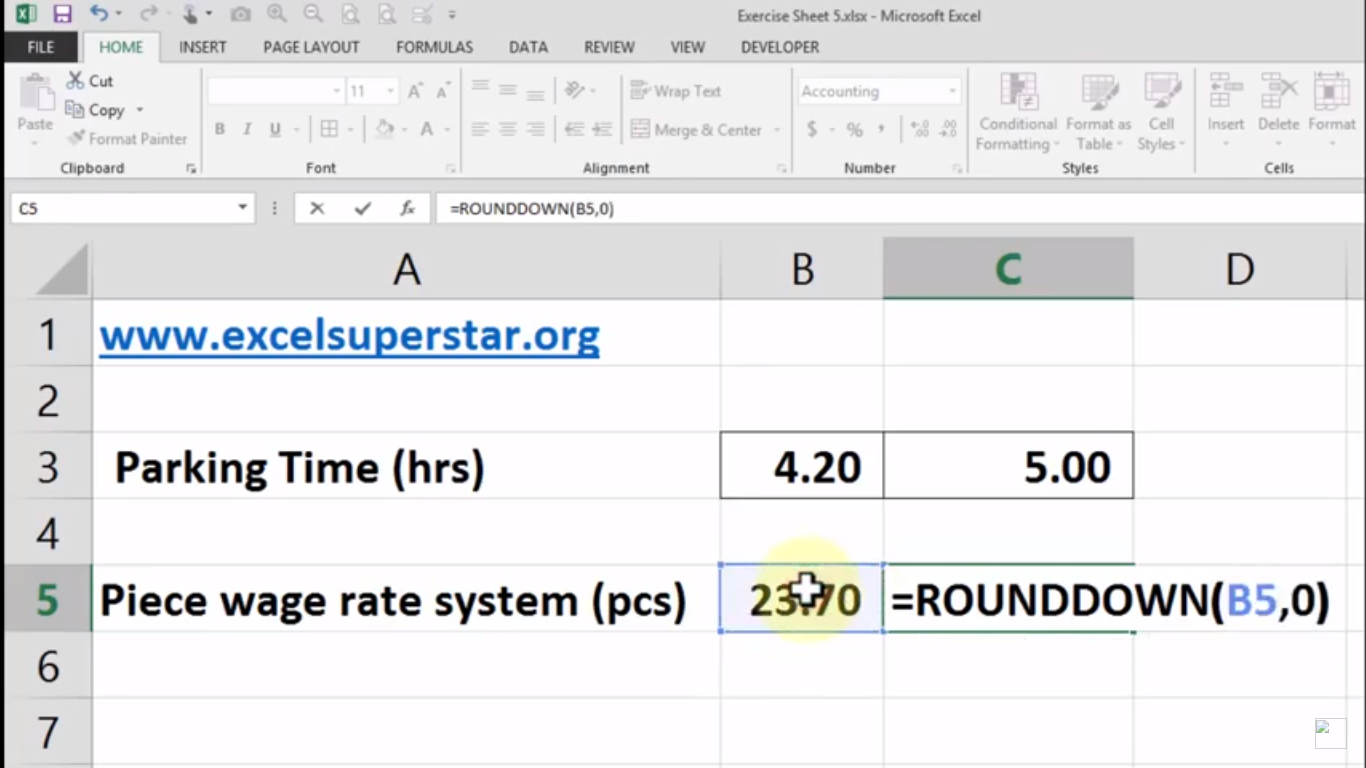# Excel ROUNDUP and ROUNDDOWN FormulaIn this tutorial, we will learn ROUNDUP & ROUNDDOWN formula with example in Excel

## 1. ROUNDUP Formula

Example –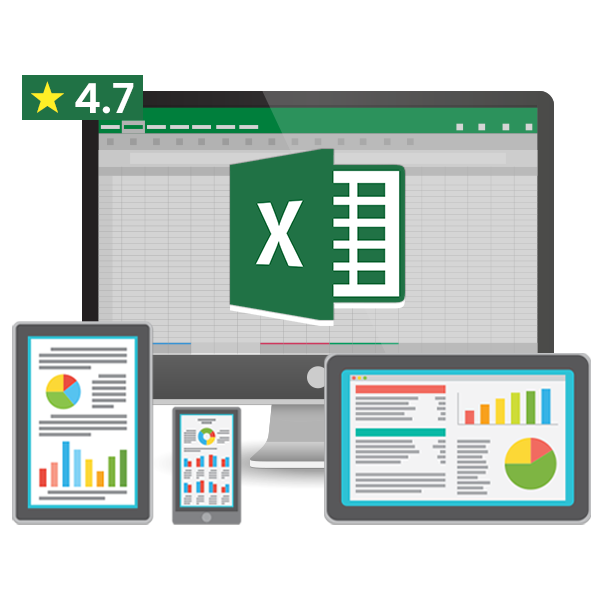50% OFF on Excel Course

• Get 2-Year Access with unlimited views of 220+ videos
• Use Coupon code - NAV50OFF & Start learning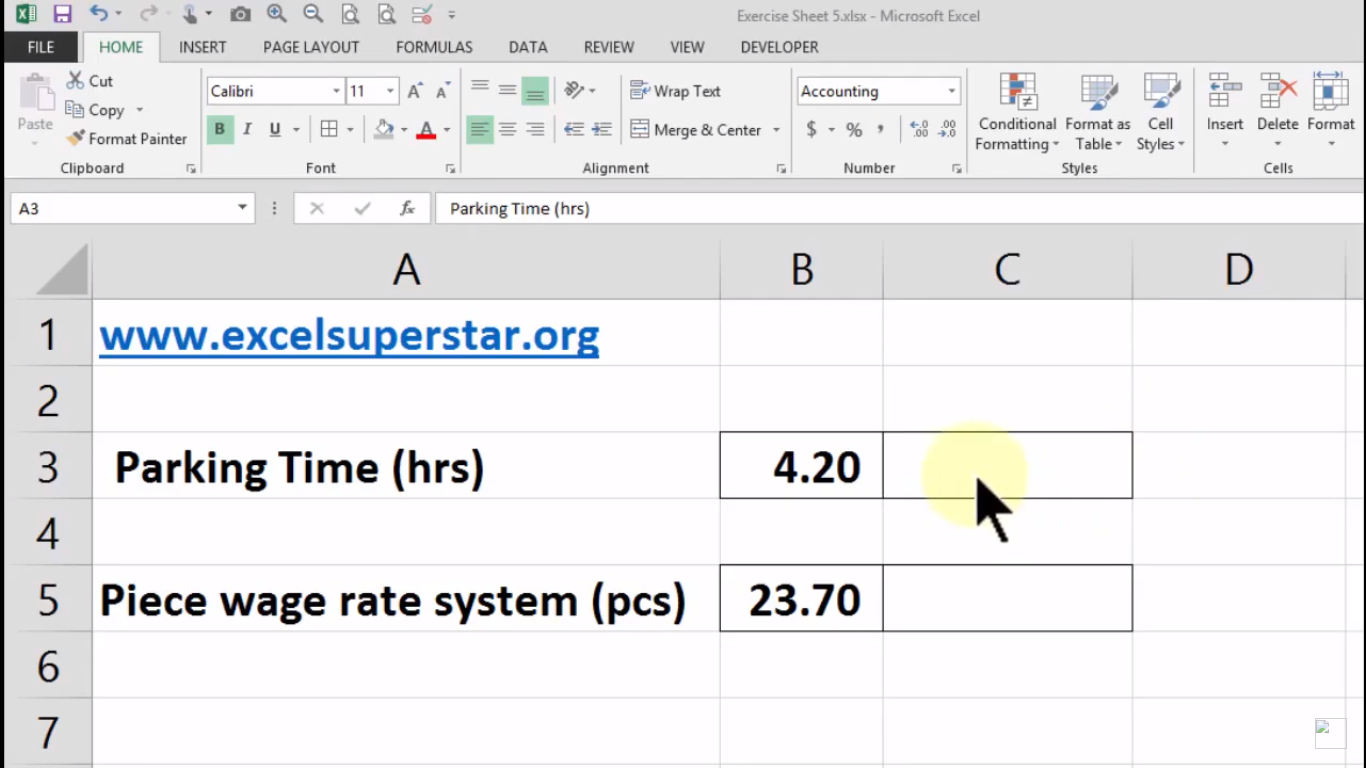Assume that you are going to a mall and you park your car in the basement. After parking, you see that the time limit of parking is 4 hours.  This means After 4 hours if one minute is extra then you have to pay for 5 hours of parking. So here they have applied ROUNDUP Formula in Excel to calculate the amount of time they have to pay for parking

1. Press =ROUNDUP and open the bracket which becomes =ROUNDUP(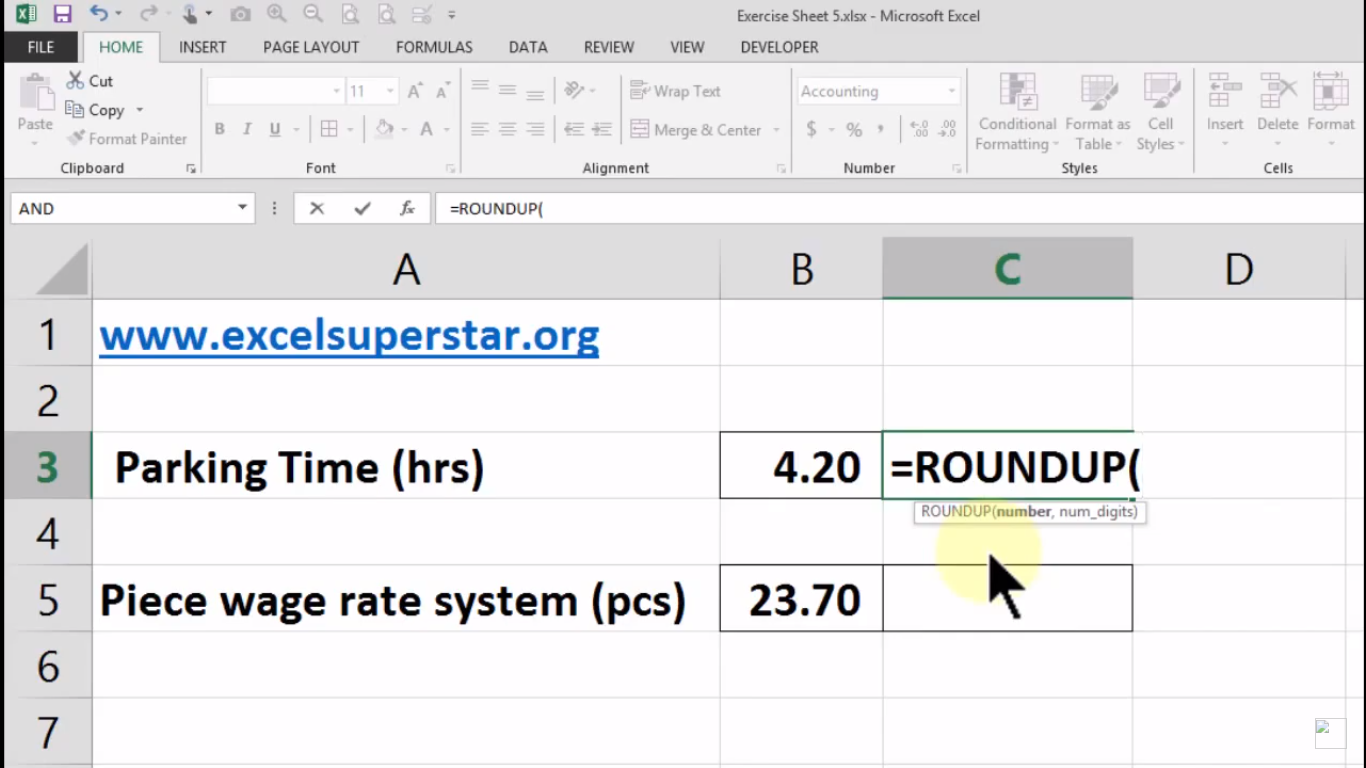2. Press Tab Key after opening the bracket

3. Choose the numbered cell and apply comma (,) so the formula will be =ROUNDUP(B3,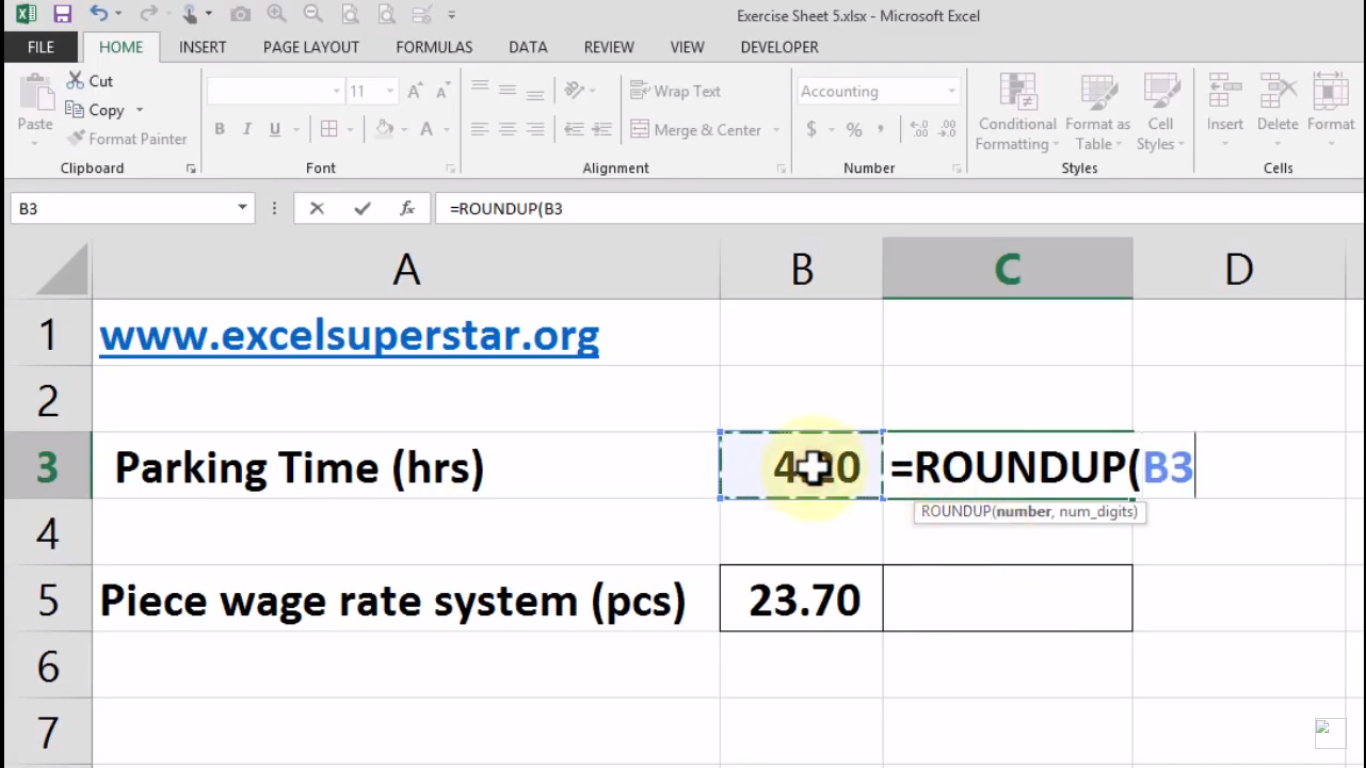4. Write 0 and close the bracket so the formula will be =ROUNDUP(B3,0)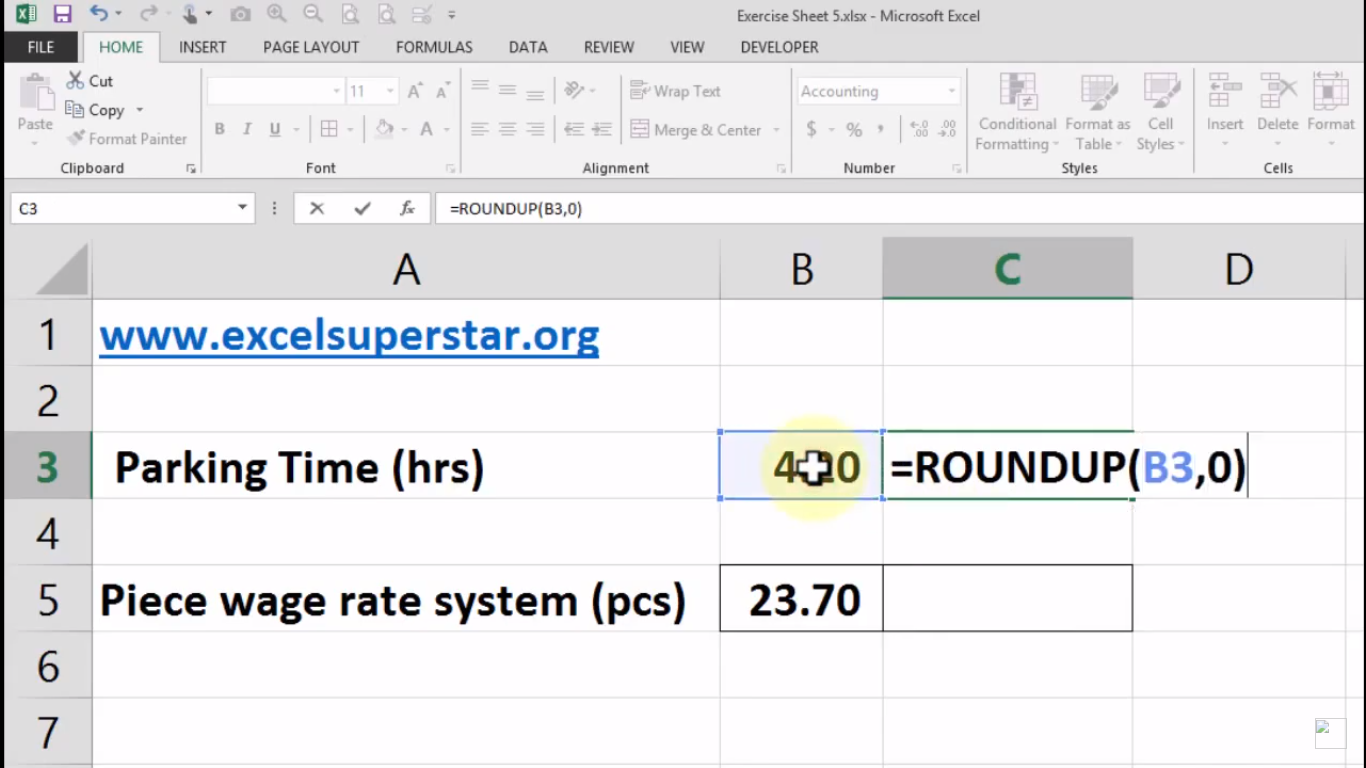Note – 0 means we don’t want any decimal which means either 4 or 5 while applying the ROUNDUP Formula

5. Lastly, press Enter and you will get your answer as 5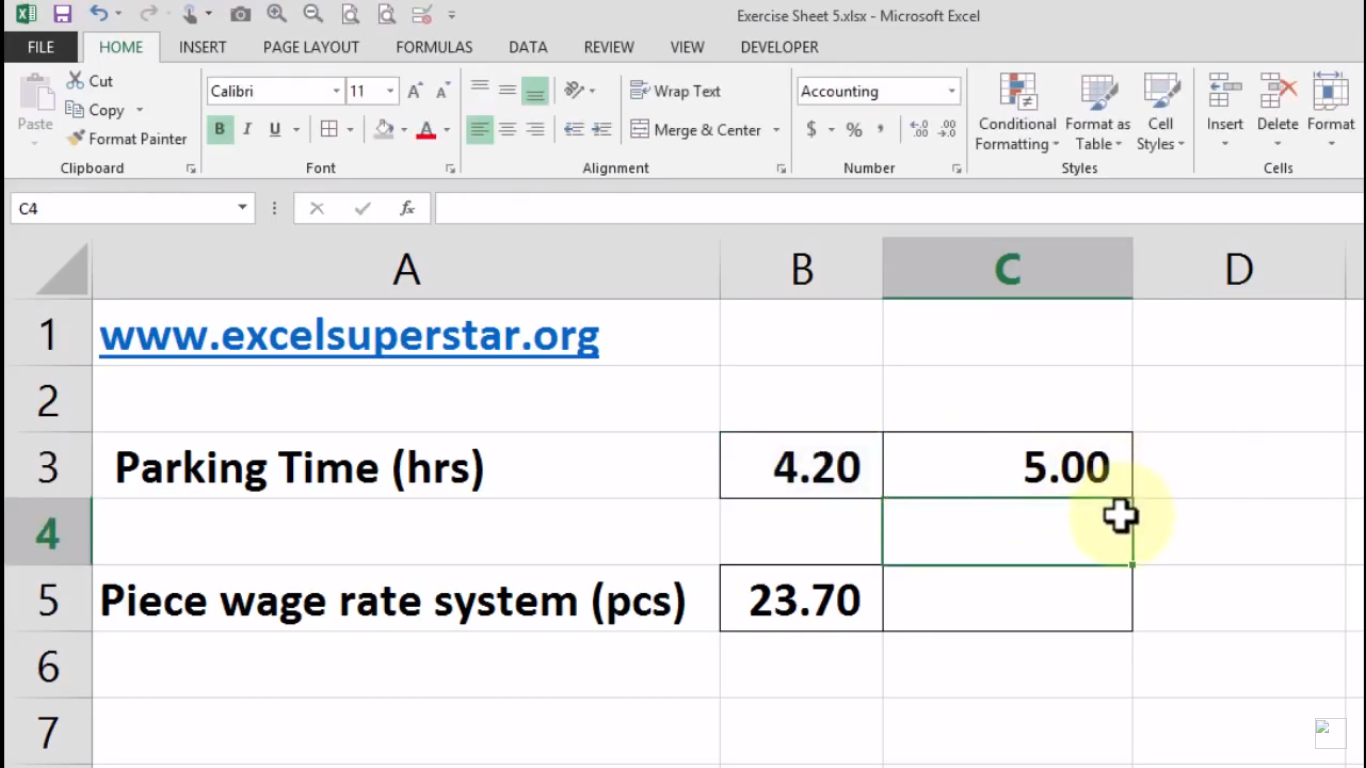## 2. ROUNDDOWN Formula

Example –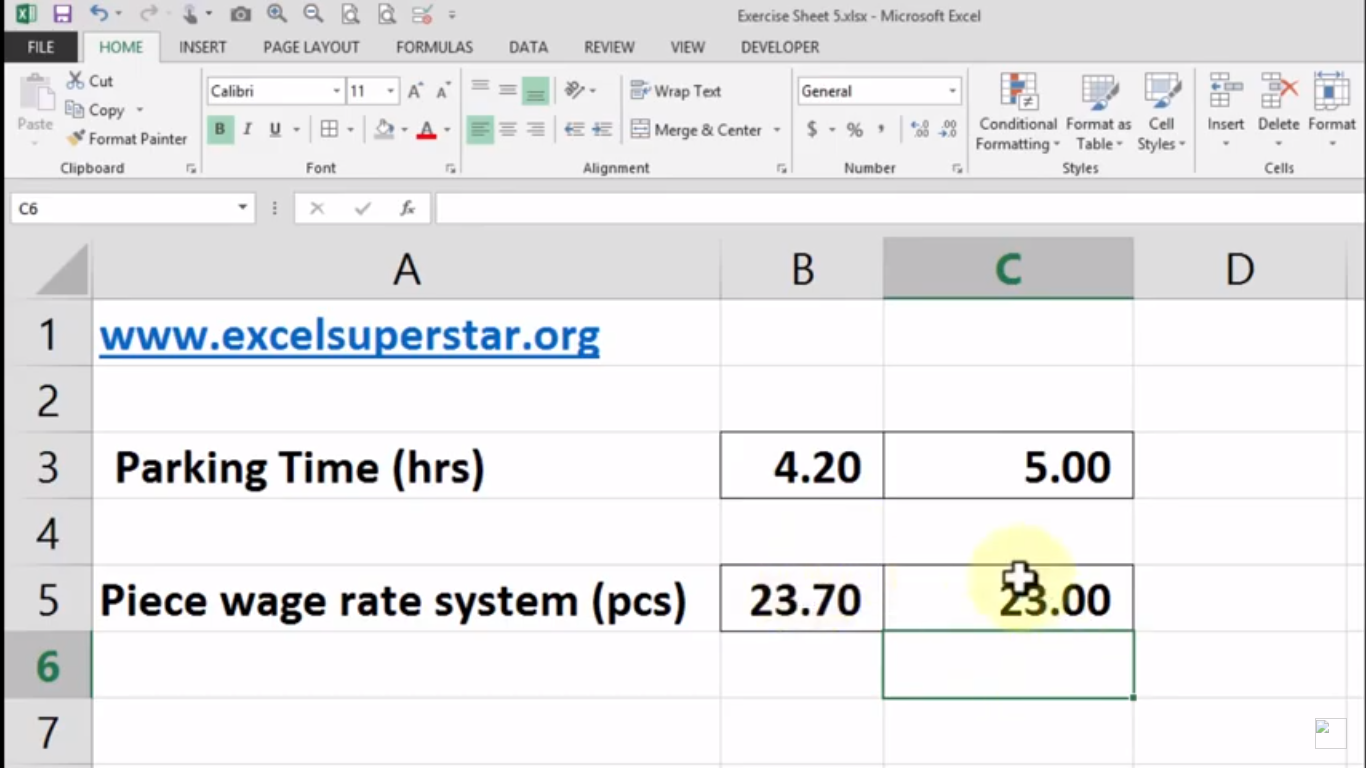In many factories, the piece wage rate system is applied which means the payment will get according to the number of pieces you have completed in a day. So here they have almost completed the product but not produced the final product so the number is 23.70.

So here they have applied ROUNDDOWN Formula in Excel to see how much money you will get for the product. Follow the steps given below:

1.Press =ROUNDDOWN and open the bracket which becomes =ROUNDDOWN(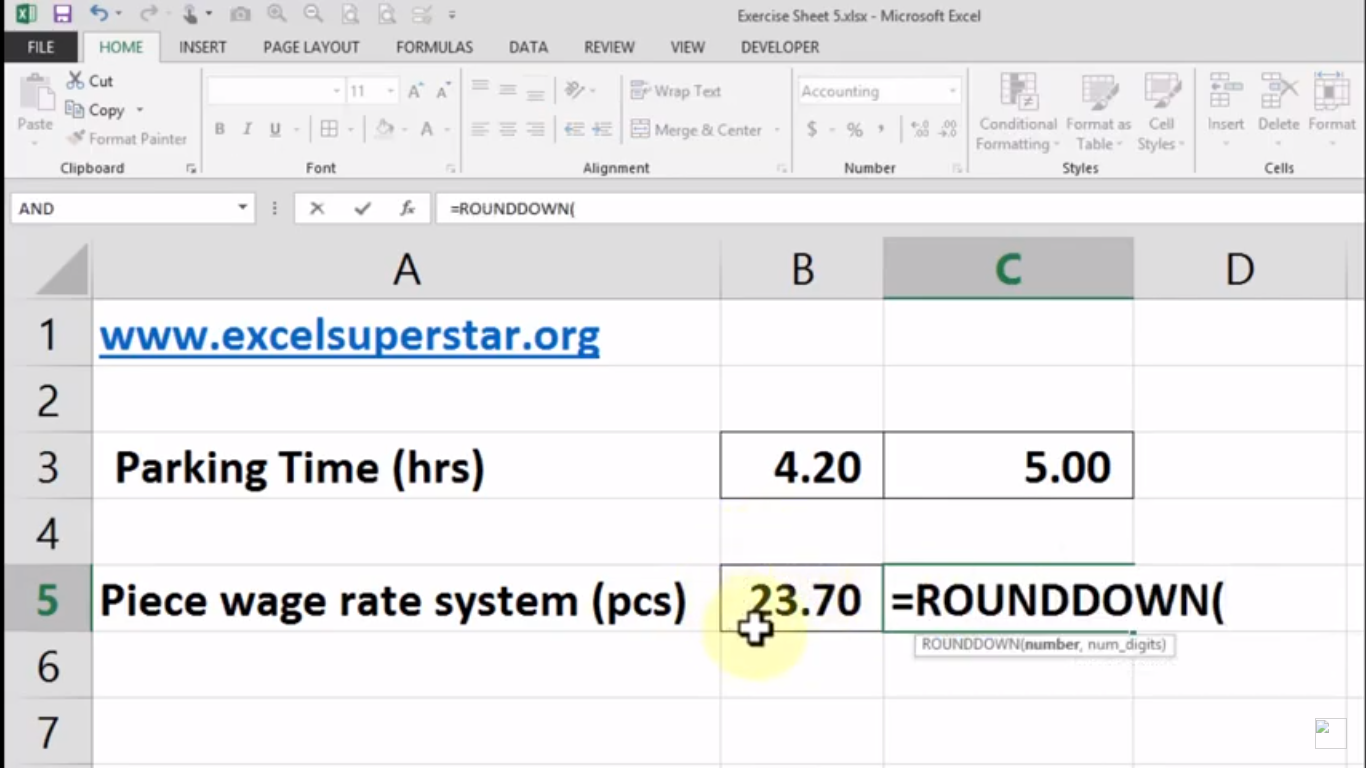2. Press Tab Key after opening the bracket

3. Choose the numbered cell and apply comma (,) so the formula will be =ROUNDDOWN(B5,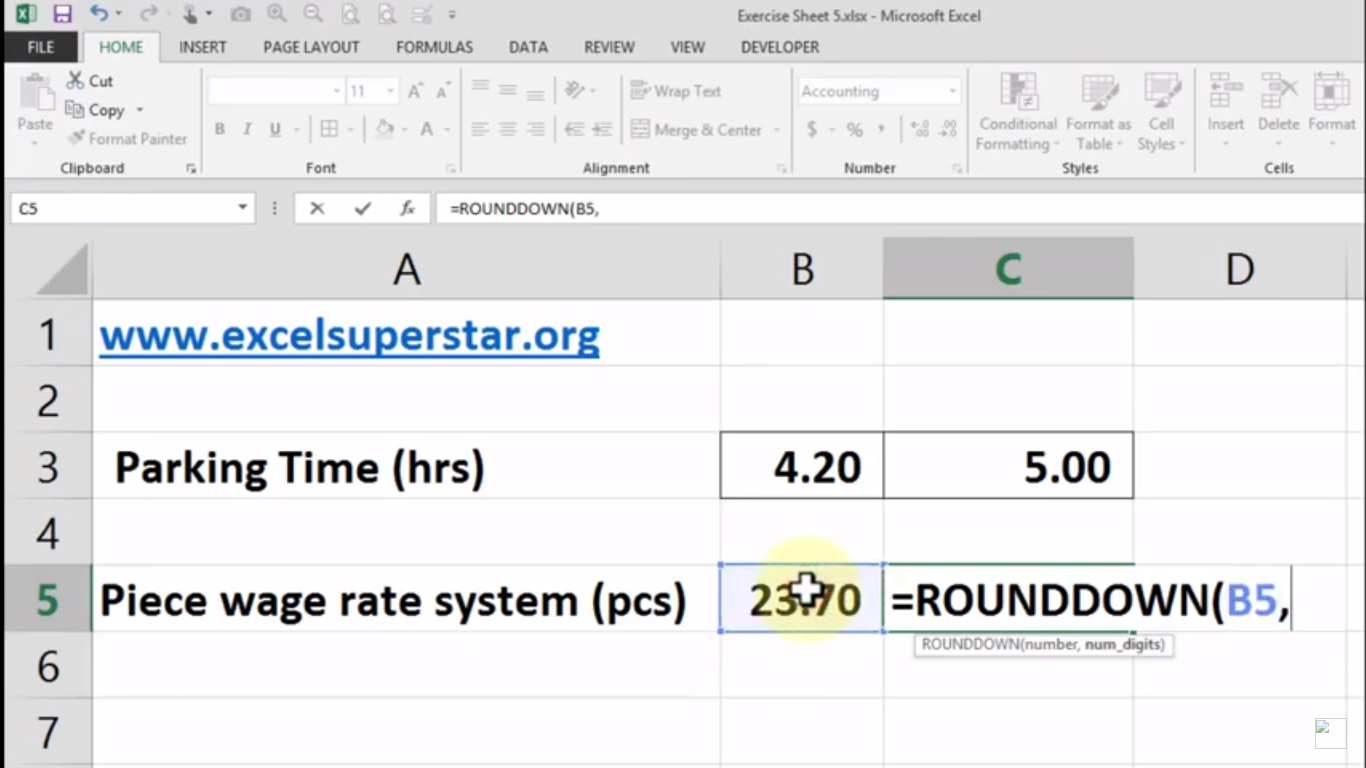4. Write 0 and close the bracket so the formula will be =ROUNDDOWN(B5,0)